# Identifying extremes and latent regimes with glmmfields

#### 2019-05-22

Here we will use the bayesdfa package to fit dynamic factor analysis (DFA) model to simulated time series data. In addition to working through an example of DFA for multivariate time series, we’ll apply bayesdfa routines for fitting hidden Markov models (HMMs) to the estimated trends to identify latent regimes. Most of the core functions of the package are included here, including fit_dfa() and find_dfa_trends for fitting DFA models, plot_trends(), plot_fitted() and plot_loadings() for plotting estimates, find_swans() for flagging extremes, fit_regimes() and find_regimes() for fitting HMM models, and plot_regimes() for plotting HMM output.

library(bayesdfa)
library(ggplot2)
library(dplyr)
library(rstan)

## DFA model with no extreme events

First, let’s simulate some data. We will use the built-in function sim_dfa(), but normally you would start with your own data. We will simulate 20 data points from 4 time series, generated from 2 latent processes. For this first dataset, the data won’t include extremes, and loadings will be randomly assigned (default).

set.seed(1)
sim_dat <- sim_dfa(
num_trends = 2,
num_years = 20,
num_ts = 4
)
matplot(t(sim_dat$y_sim), type = "l", ylab = "Response", xlab = "Time" )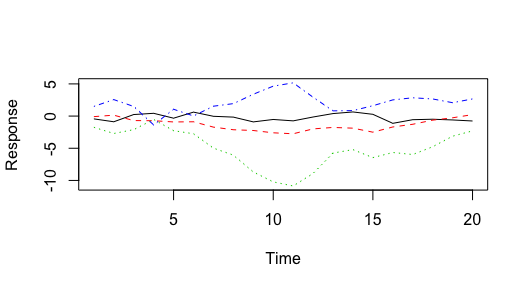Simulated data, from a model with 2 latent trends and no extremes. Next, we’ll fit a 1-trend, 2-trend, and 3-trend DFA model to the simulated time series using the fit_dfa() function. Starting with the 1-trend model, we’ll estimate the posterior distributions of the trends and loadings. Note that this example uses 1 MCMC chain and 200 iterations — for real examples, you’ll want to use more (say 4 chains, 5000 iterations). f1 <- fit_dfa( y = sim_dat$y_sim, num_trends = 1, zscore = TRUE,
iter = 200, chains = 1, thin = 1
)

Convergence of DFA models can be evaluated with our is_converged() function. This function takes a fitted object, and specified threshold argument representing the maximum allowed Rhat value (default = 1.05). The convergence test isn’t that useful for a model with such a short number of iterations, but is called with

is_converged(f1, threshold = 1.05)
##  TRUE

Before we extract the trends from the model, we need to rotate the loadings matrix and trends. By default we use the varimax rotation, implemented in the rotate_trends() function. An optional argument is the conf_level argument, which calculates the specified confidence (credible) interval of the estimates (by default, this is set to 0.95).

r <- rotate_trends(f1)

The rotated object has several quantities of interest, including the mean values of the trends “trends_mean” and loadings “Z_rot_mean”,

names(r)
##  "Z_rot"         "trends"        "Z_rot_mean"    "Z_rot_median"
##  "trends_mean"   "trends_median" "trends_lower"  "trends_upper"

We can then plot the trends and intervals, with

plot_trends(r)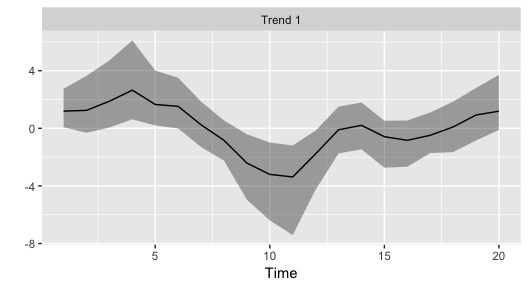Estimated trend and 95% CI for a 1-trend DFA model applied to simulated data.

We can also plot the estimated loadings (we’ll show that plot for the more complicated 2-trend model below because it’s not as interesting for the 1-trend model) and the fitted values. To plot the fitted values from the 1-trend model, we’ll use the plot_fitted() function (predicted values can also be returned without a plot, with the predicted()) function.

We can then plot the trends and intervals, faceting by time series, with

plot_fitted(f1)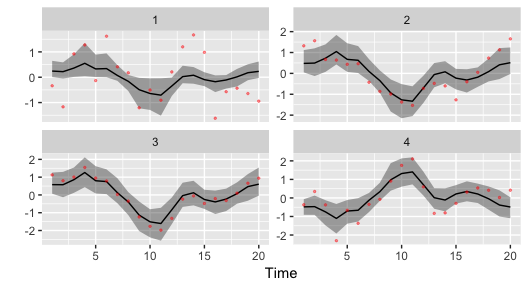Model predicted values from the 1-trend DFA model applied to simulated data.

Moving to a more complex model, we’ll fit the 2-trend and 3-trend models. All other arguments stay the same as before,

f2 <- fit_dfa(
y = sim_dat$y_sim, num_trends = 2, zscore = TRUE, iter = 200, chains = 1, thin = 1 ) r2 <- rotate_trends(f2) f3 <- fit_dfa( y = sim_dat$y_sim, num_trends = 3, zscore = TRUE,
iter = 200, chains = 1, thin = 1
)
r3 <- rotate_trends(f3)

The fits from the 2-trend model look considerably better than that from the 1-trend model,

plot_fitted(f2)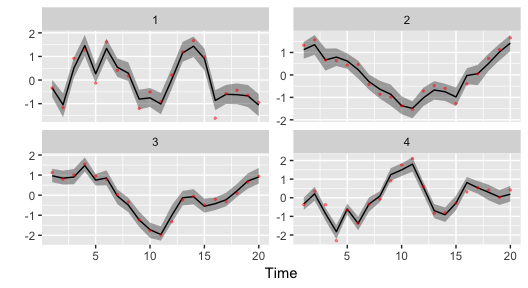Model predicted values from the 2-trend DFA model applied to simulated data.

The loadings from the 1-trend model aren’t as interesting because for a 1-trend model the loadings are a 1-dimensional vector. For the 2 trend model, there’s a separate loading of each time series on each trend,

round(r2$Z_rot_mean, 2) ## [,1] [,2] ## [1,] 0.78 -0.09 ## [2,] -0.07 0.60 ## [3,] 0.30 0.55 ## [4,] -0.69 -0.26 These loadings can also be plotted with the plot_loadings() function. This shows the distribution of the densities as violin plots, with color proportional to being different from 0. plot_loadings(r2)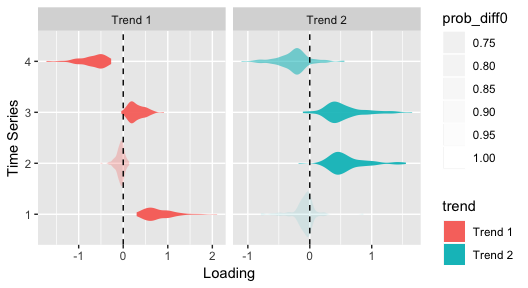Estimated loadings from the 2-trend DFA model. Finally, we might be interested in comparing some measure of model selection across these models to identify whether the data support the 1-trend, 2-trend, or 3-trend models. The Leave One Out Information Criterion can be calculated with the loo() function, for example the LOOIC for the 1-trend model can be accessed with loo1 <- loo(f1) loo1$estimates
##           Estimate        SE
## elpd_loo -90.77104  5.221621
## p_loo     10.89671  1.303337
## looic    181.54208 10.443242

where 181.5420834 is the estimate and 10.4432421 is the standard error.

As an alternative to fitting each model individually as we did above, we also developed the find_dfa_trends() to automate fitting a larger number of models. In addition to evaluating different trends, this function allows the user to optionally evaluate models with normal and Student-t process errors, and alternative variance structures (observation variance of time series being equal, or not). For example, to fit models with 1:5 trends, both Student-t and normal errors, and equal and unequal variances, the call would be

m <- find_dfa_trends(
y = s$y_sim, iter = 180, kmin = 1, kmax = 5, chains = 1, compare_normal = TRUE, variance = c("equal", "unequal") ) ## DFA model with extreme events In this example, we’ll simulate data with an extreme anomaly. As before, this will be 20 data points from 4 time series, generated from 2 latent processes. The sim_dfa() function’s arguments extreme_value and extreme_loc allow the user to specify the magnitude of the extreme (as an additive term in the random walk), and the location of the extreme (defaults to the midpoint of the time series). Here we’ll include an extreme value of 6, set.seed(1) sim_dat <- sim_dfa( num_trends = 2, num_years = 20, num_ts = 4, extreme_value = 6 ) Plotting the data shows the anomaly occurring between time step 9 and 10, matplot(t(sim_dat$y_sim), type = "l", ylab = "Response", xlab = "Time")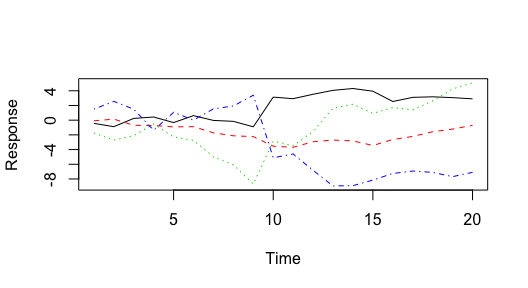Simulated data, from a model with 2 latent trends and an extreme in the midpoint of the time series.

Though the plot is a little more clear if we standardize the time series first,

matplot(scale(t(sim_dat$y_sim)), type = "l", ylab = "Response", xlab = "Time")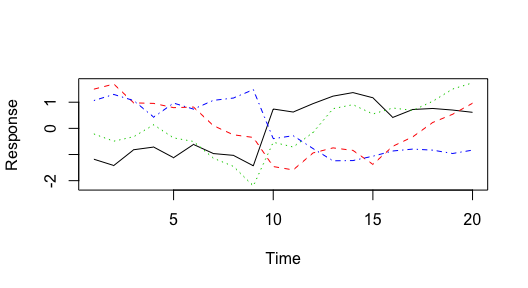Simulated data (z-scored), from a model with 2 latent trends and an extreme in the midpoint of the time series. Instead of fitting a model with normal process deviations, we may be interested in fitting the model with Student-t deviations. We can turn on the estimation of nu with the estimate_nu argument. [Alternatively, nu can also be fixed a priori by setting the argument nu_fixed]. Here’s the code for a 2-trend model with Student-t deviations, t2 <- fit_dfa( y = sim_dat$y_sim, num_trends = 2, zscore = TRUE,
iter = 200, chains = 1, thin = 1, estimate_nu = TRUE
)

Again we have to rotate the trends before plotting,

r <- rotate_trends(t2)
plot_trends(r)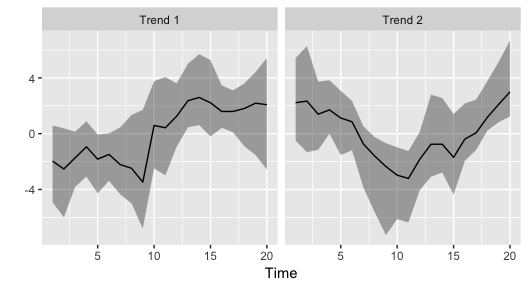Estimated trends, from a model with 2 latent trends Student-t deviations.

plot_loadings(r)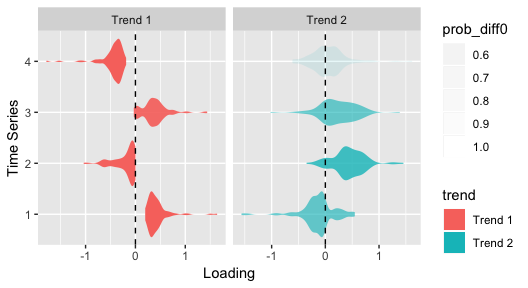One way to look for extremes is using the find_swans() function, which evaluates the probability of observing a deviation in the estimated trend (or data) greater than what is expected from a normal distribution. This function takes a threshold argument, which specifies the cutoff. For example, to find extremes greater than 1 in 1000 under a normal distribution, the function call is

find_swans(r, plot = FALSE, threshold = 1 / 1000)

Setting plot to TRUE also creates a time series plot that flags these values.

We can also look at the estimated nu parameter, which shows some support for using the Student-t distribution (values greater than ~ 30 lead to similar behavior as a normal distribution),

summary(rstan::extract(t2$model, "nu")[]) ## V1 ## Min. : 5.807 ## 1st Qu.:11.030 ## Median :16.515 ## Mean :19.342 ## 3rd Qu.:24.094 ## Max. :60.323 ## Applying Hidden Markov Models to identify latent regimes Finally, we might be interested in evaluating evidence for the estimated trends changing between multiple regimes. We’ve implemented HMMs in the functions fit_regimes() and find_regimes(). fit_regimes fits a HMM with a pre-specified number of latent states, while find_regimes() loops over a range of models so that support can be evaluated with LOOIC. We’ll illustrate an example of the fit_regimes() function. Note that in the current version of the package, this has to be applied to each trend separately. Also, uncertainty estimates from the DFA trends may also be included as data (instead of estimated) – this may be particularly useful for datasets with lots of missing values in portions of the time series (where the uncertainty balloons up). Applying the 2-regime model to the first trend, reg_mod <- fit_regimes( y = r$trends_mean[1, ],
sds = (r$trends_upper - r$trends_mean)[1, ] / 1.96,
n_regimes = 2,
iter = 200, chains = 1
)

In addition to getting diagnostics and quantities like LOOIC out of the reg_mod object, we can plot the estimated states.

plot_regime_model(reg_mod)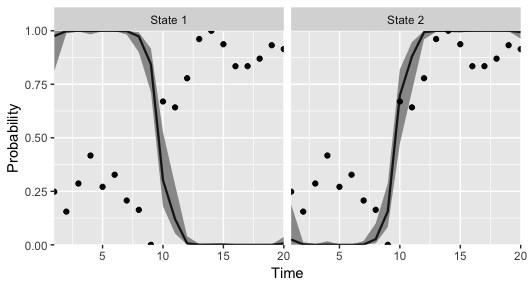Estimated regimes, from a HMM model applied to the first trend of a 2-trend DFA model with Student-t deviations.

In this case, there might be some support for the 2-regime model. Sometimes (but not in this example) label switching makes the plots a little challenging to interpret, and the labels need to be reversed. We’ve included the argument flip_regimes to help with this,

plot_regime_model(reg_mod, flip_regimes = TRUE)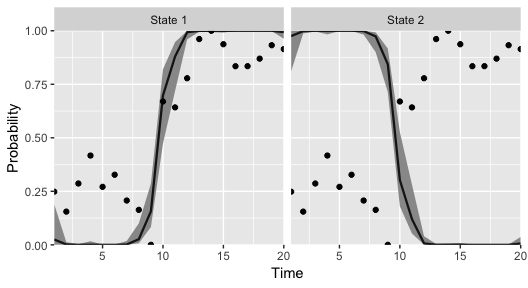Estimated regimes (after flipping), from a HMM model applied to the first trend of a 2-trend DFA model with Student-t deviations.